International
Tables for
Crystallography
Volume B
Reciprocal space
Edited by U. Shmueli

International Tables for Crystallography (2006). Vol. B. ch. 2.1, pp. 203-204   | 1 | 2 |

## Section 2.1.8.1. General representations of p.d.f.'s ofby Fourier series

U. Shmuelia* and A. J. C. Wilsonb

aSchool of Chemistry, Tel Aviv University, Tel Aviv 69 978, Israel, and bSt John's College, Cambridge, England
Correspondence e-mail:  ushmueli@post.tau.ac.il

#### 2.1.8.1. General representations of p.d.f.'s ofby Fourier series

| top | pdf |

We assume, as before, that (i) the atomic phase factors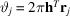[cf. equation (2.1.1.2)] are uniformly distributed on (0–2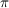) and (ii) the atomic contributions to the structure factor are independent. For a centrosymmetric space group, with the origin chosen at a centre of symmetry, the random variable is the (real) normalized structure factor E and its bounds are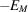and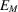, where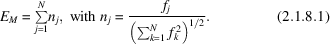Here,is the maximum possible value of E andis the conventional scattering factor of the jth atom, including its temperature factor. The p.d.f.,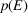, can be non-zero in the range (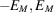) only and can thus be expanded in the Fourier series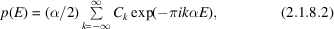where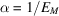. Only the real part ofis relevant. The Fourier coefficients can be obtained in the conventional manner by integrating over the range (),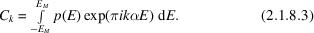Since, however,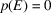for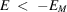and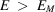, it is possible and convenient to replace the limits of integration in equation (2.1.8.3)by infinity. Thus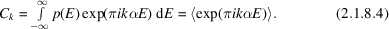Equation (2.1.8.4)shows that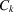is a Fourier transform of the p.d.f.and, as such, it is the value of the corresponding characteristic function at the point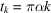[i.e.,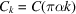, where the characteristic function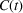is defined by equation (2.1.4.1)]. It is also seen thatis the expected value of the exponential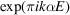. It follows that the feasibility of the present approach depends on one's ability to evaluate the characteristic function in closed form without the knowledge of the p.d.f.; this is analogous to the problem of evaluating absolute moments of the structure factor for the correction-factor approach, discussed in Section 2.1.7. Fortunately, in crystallographic applications these calculations are feasible, provided individual isotropic motion is assumed. The formal expression for the p.d.f. of, for any centrosymmetric space group, is therefore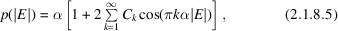where use is made of the assumption that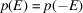, and the Fourier coefficients are evaluated from equation (2.1.8.4).

The p.d.f. offor a noncentrosymmetric space group is obtained by first deriving the joint p.d.f. of the real and imaginary parts of E and then integrating out its phase. The general expression for E is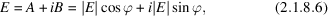whereis the phase of E. The required joint p.d.f. is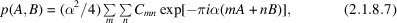and introducing polar coordinates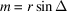and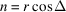, where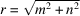and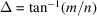, we have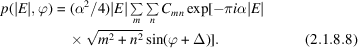Integrating out the phase, we obtain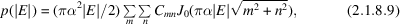where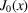is the Bessel function of the first kind (e.g. Abramowitz & Stegun, 1972). This is a general form of the p.d.f. offor a noncentrosymmetric space group. The Fourier coefficients are obtained, similarly to the above, as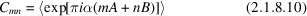and the average in equation (2.1.8.10), just as that in equation (2.1.8.4), is evaluated in terms of integrals over the appropriate trigonometric structure factors. In terms of the characteristic function for a joint p.d.f. of A and B, the Fourier coefficient in equation (2.1.8.10)is given by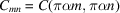.

We shall denote the characteristic function by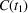if it corresponds to a Fourier coefficient of a Fourier series for a centrosymmetric space group and by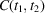or by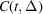, where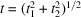and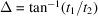, if it corresponds to a Fourier series for a noncentrosymmetric space group.

### ReferencesAbramowitz, M. & Stegun, I. A. (1972). Handbook of mathematical functions. New York: Dover.Google Scholar